# Identifying numeric obfuscation

Published: 2018-08-26
Last Updated: 2018-08-26 10:56:21 UTC
by Didier Stevens (Version: 1)
0 comment(s)

I was asked for help with the analysis of a malicious script.

The solution is easy: the script contains another script, encoded with numbers using a simple substitution cipher (shift 12).

The problem is identifying that numeric obfuscation is used, and figuring out which one exactly.

One way to try to identify it, of course, is just to look at the script: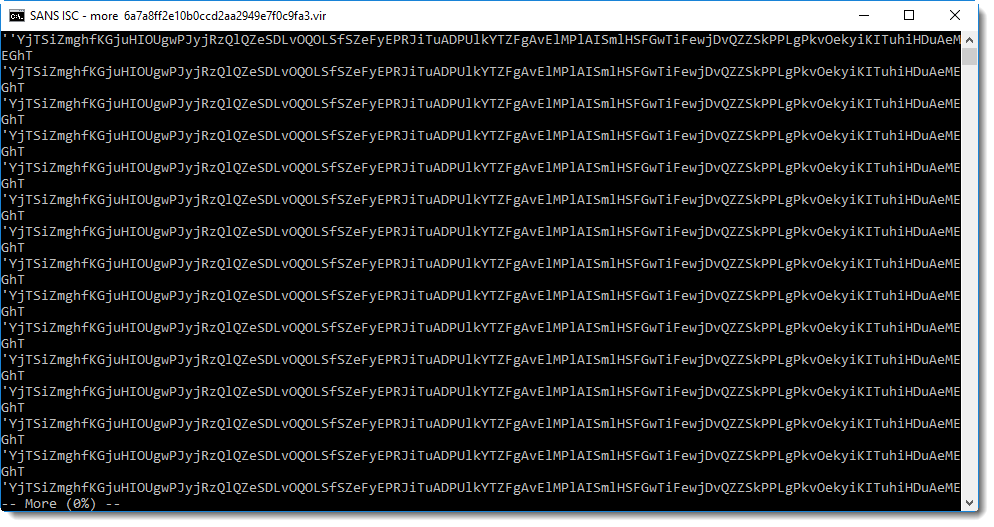Scrolling down, you will find this: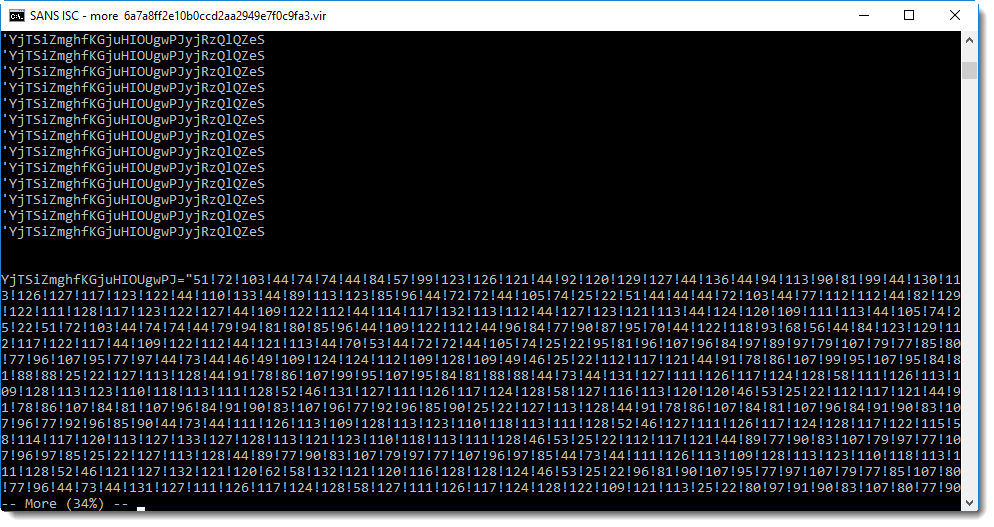That's clearly a string with a large amount of numbers separated by an exclamation mark (!). This is a clear indicator of numeric obfuscation in malicious scripts: a list of numbers.

Now you need to convert these numbers to ASCII characters. And maybe first apply a mathematical transformation on each number (depending on the type of obfuscation).

I have a tool to help with this: numbers-to-string.py. This tool reads a text file, extracts numbers per line, performs an optional calculation on each number, and then converts them to ASCII.

There is a new option in this tool, to perform a simple statistical analysis. This is done with option -S: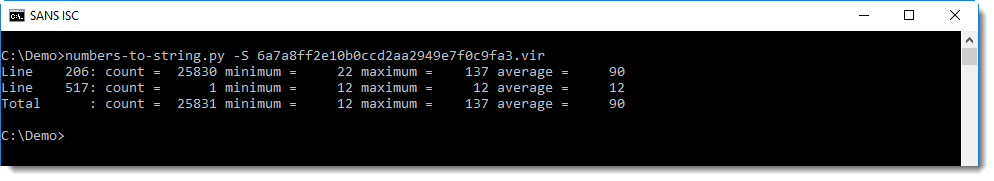With this information, you know that:

• on line 206, 25830 numbers were found, ranging between 22 and 137, and with an average value of 90.
• on line 517, a single number was found: 12

All numbers between 22 and 126 can be converted to an ASCII character, and numbers between 127 to 137 to an extended ASCII character (depending on the code page).

Because we will end up with extended ASCII characters if we just convert the numbers to a character, it's probably that we need to perform a mathematical operation on each number, to end up with just ASCII characters.

You can first try without mathematical operation (""), like this: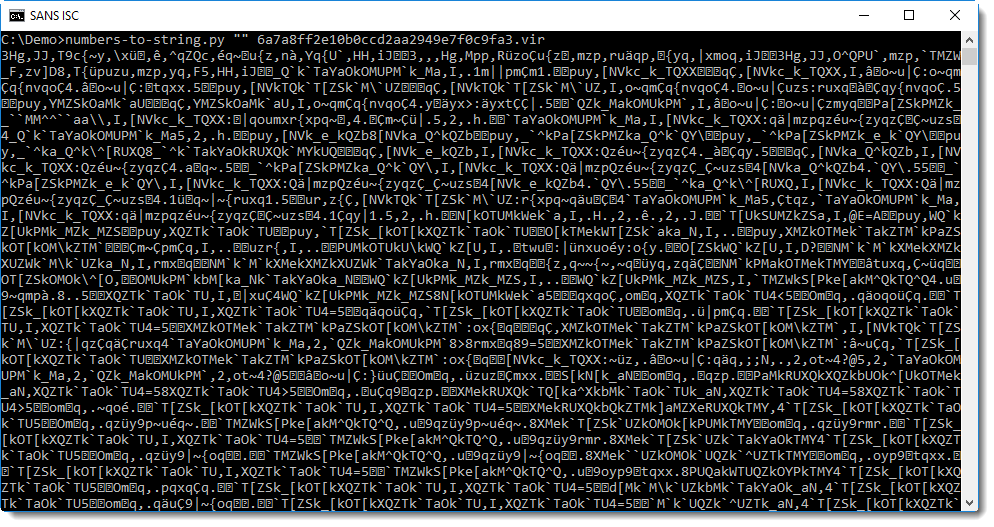It's clear that this is not what you are looking for. This does not look like a script. But nevertheless, this output provides a bit of information: that all numbers can be converted to a character. If this would not be possible, then numbers-to-string would not output anything for that line.

The next step is to figure out what mathematical operation has to be performed. This too can be done with just trial-and-error, by starting with the most simple operation: adding or subtracting a constant number.

• n + c
• n - c

Where n is the number found in the obfuscated script, and c is the constant to add or subtract. But what constant should you use?

Remember the output of the statiscal analysis of the script: there was a second line, with a single number: 12.

So first try with 12:

• n + 12
• n - 12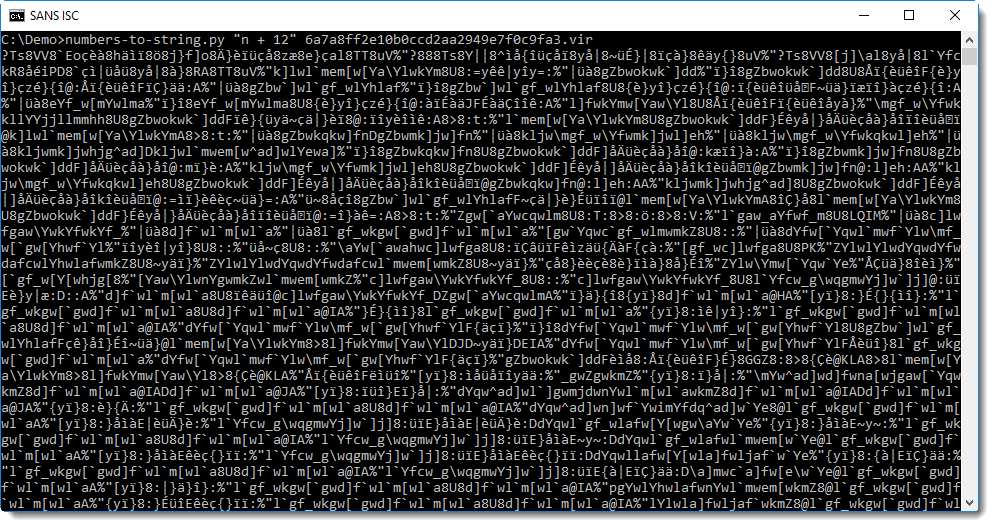That's no improvement. Try with "n - 12" now:That's clearly a script: it's a variant of the H-worm. This one connects to C2 shkis[.]publicvm[.]com on port 83 via HTTP. It polls the C2 about every 5 seconds with a POST command using path /is-ready to indicate that it is ready to execute commands.

In my experience, simple numeric obfuscation like this sample appears frequently. By looking more closely at the statistical results, one could also deduce that the operation is a subtraction: because there are numbers larger than 126, and for ASCII, the largest printable character is 126 (~).

XORSearch can also help to identify the mathematical operation to be performed. I'll probably cover this in another diary entry.

Didier Stevens
Senior handler
Microsoft MVP
blog.DidierStevens.com DidierStevensLabs.com

0 comment(s)